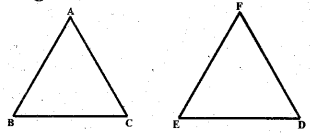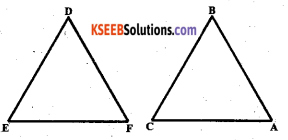# KSEEB Solutions for Class 7 Maths Chapter 7 Congruence of Triangles Ex 7.1

Students can Download Chapter 7 Congruence of Triangles Ex 7.1, Question and Answers, Notes Pdf, KSEEB Solutions for Class 7 Maths, Karnataka State Board Solutions help you to revise complete Syllabus and score more marks in your examinations.

## Karnataka State Syllabus Class 7 Maths Chapter 7 Congruence of Triangles Ex 7.1

Question 1.
Complete the following statements :
a) Two line segments – are congruent if ___.
Solution:
They have the same measure, (length)

b) Among two congruent angles, one has a measure of 70°; the measure of the other angle is ___
Solution:
70°

c) When we write ∠A = ∠B, we actually mean ___
m < A ≅ mQuestion 2.
Give any two real-life examples for congruent shapes.
Solution:
Two Rs 10/- notes and two ten rupees coins.

Question 3.
If ∆ ABC ≅ ∆ FED under the correspondence ABC ⟷ FED, write all the corresponding congruent parts of the triangles.Solution:
The ∆ ABC ≅ ∆ FED then the corresponding vertices A and F, B and E, C and D. The corresponding sides are$\overline{\mathrm{AB}}$ and FE, BC and ED, CA, and DF. The corresponding angles are ∠A and ∠F, ∠B and ∠E, and ∠C and ∠D.Question 4.
If ∆ DEF ≅ ∆ BAC, write the part(s) of ∆ BCA that correspond to
Solution:i) ∠E
∠E ≅ ∠C,

ii) $$\overline{\mathbf{E} \mathbf{F}}$$
$$\overline{\mathbf{E} \mathbf{F}}$$ ≅ $$\overline{\mathbf{C} \mathbf{A}}$$

iii) ∠F
∠F ≅ ∠A

iv) $$\overline{\mathbf{D F}}$$
$$\overline{\mathbf{D F}}$$ ≅ $$\overline{\mathbf{B A}}$$

error: Content is protected !!